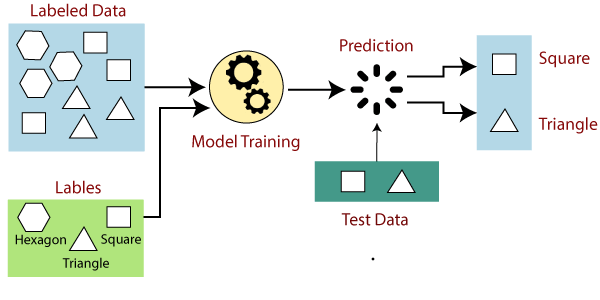# Supervised Machine Learning

Supervised learning is the types of machine learning in which machines are trained using well “labelled” training data, and on basis of that data, machines predict the output. The labelled data means some input data is already tagged with the correct output.

In supervised learning, the training data provided to the machines work as the supervisor that teaches the machines to predict the output correctly. It applies the same concept as a student learns in the supervision of the teacher.

Supervised learning is a process of providing input data as well as correct output data to the machine learning model. The aim of a supervised learning algorithm is to find a mapping function to map the input variable(x) with the output variable(y) .

In the real-world, supervised learning can be used for Risk Assessment, Image classification, Fraud Detection, spam filtering , etc.

## How Supervised Learning Works?

In supervised learning, models are trained using labelled dataset, where the model learns about each type of data. Once the training process is completed, the model is tested on the basis of test data (a subset of the training set), and then it predicts the output.

The working of Supervised learning can be easily understood by the below example and diagram:Suppose we have a dataset of different types of shapes which includes square, rectangle, triangle, and Polygon. Now the first step is that we need to train the model for each shape.

• If the given shape has four sides, and all the sides are equal, then it will be labelled as a Square .
• If the given shape has three sides, then it will be labelled as a triangle .
• If the given shape has six equal sides then it will be labelled as hexagon .

Now, after training, we test our model using the test set, and the task of the model is to identify the shape.

The machine is already trained on all types of shapes, and when it finds a new shape, it classifies the shape on the bases of a number of sides, and predicts the output.

## Steps Involved in Supervised Learning:

• First Determine the type of training dataset
• Collect/Gather the labelled training data.
• Split the training dataset into training dataset, test dataset, and validation dataset .
• Determine the input features of the training dataset, which should have enough knowledge so that the model can accurately predict the output.
• Determine the suitable algorithm for the model, such as support vector machine, decision tree, etc.
• Execute the algorithm on the training dataset. Sometimes we need validation sets as the control parameters, which are the subset of training datasets.
• Evaluate the accuracy of the model by providing the test set. If the model predicts the correct output, which means our model is accurate.

n supervised learning, the training data that you feed into your machine learning algorithm also contains the desired solutions called labels.

Take, for instance, the example of a spam filter. The task is to classify the new emails as spam or ham(not spam).

You will provide the data to your machine learning algorithm which will contain the examples of both types of mails i.e spam and ham. Each of the emails would have a label associated with it, telling whether the given email is a spam or a ham. Now, your algorithm will learn according to this dataset, whether a new email would be a spam or a ham.